#### Ionic Bonding, Chemistry tutorial

Introduction

When bonding take place, the consequential molecule or compound has a lower energy than its constituent atoms. Bonding is accomplished through redistributing the valence (or bonding) electrons. In ionic bonding, this redistribution takes place through the atoms shifting one or more electrons. The term ionic bond describes the electrostatic attraction of 2 oppositely charged ions in a crystalline lattice. Molecules that consist of charged ions via opposite charges are termed ionic molecules. Such ionic compounds are commonly solids through high melting points and conduct electrical current. Ionic compounds are usually shaped from metal and non-metal elements.

For instance, we are familiar through the fairly benign unspectacular behaviour of ordinary white crystalline table salt (NaCl). Salt consists of positive sodium ions (Na+) and negative chloride ions (Cl-). On the other hand the element sodium is a silvery gray metal composed of neutral atoms that react vigorously through water or air. Chlorine as an element is a neutral greenish-yellow, poisonous, diatomic gas (Cl2).

The major principle to remember is that ions are entirely diverse in physical and chemical properties from the neutral atoms of the element. The details of the + and - charges on ions is very important as it conveys a definite meaning. Whereas elements are neutral in charge, IONS have either a positive or negative charge depending upon whether there is an excess of protons (positive ion) or excess of electrons (negative ion).

Basic Principles of Bonding

What are molecules made from? They are made from atoms, which are themselves made from nuclei and electrons. Such building blocks take an electrical charge: nuclei are positively charged, and electrons are negatively charged. The nuclei themselves are made up of (positively charged) protons and (neutral) neutrons. This is all summarized on the given picture: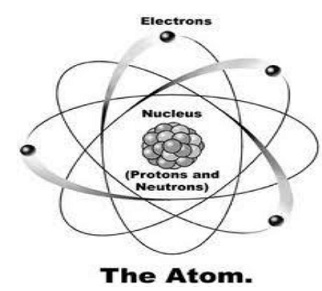Different kinds of atom have diverse no of protons, neutrons, and electrons. For instance, carbon atoms have six protons, six neutrons, and six electrons.

Charged species interact through each other: like charges (+ and + or - and -) repel each other, opposite charges (- and +) attract each other. This familiar principle from physics is summarized via Coulomb's law:

F= 1/4πεο (q1q2/r2)

(Here, F is the force between the 2 charges; ε0 is a constant (not important here), q1 and q2 are the values of the charges involved, and r is the distance between them.)

This force between charged species is middle to all of chemistry, and in particular to all the kinds of bonding we will discuss.

1st, it clarifies how atoms hold mutually: the negatively charged electrons are magnetized to the positively charged nucleus more than they are repelled through the other electrons.

There is a fine balance between the attractive force holding the electrons close to the nucleus, and the repulsive force that tends to keep electrons away from each other. The consequence of this competition between attractive and repulsive charge-charge interactions is what explains the detailed structure of atoms. The electrons in atoms tend to form into concentric shells. For the hydrogen atom, through just one electron and one proton (Z = 1), the electron sits in the 1st shell, as shown here: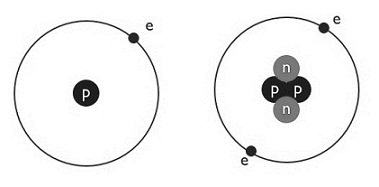Hydrogen Atom (z=1)                        helium Atom (z=2)

The Helium atom has 2 protons, two neutrons and 2 electrons (that is Z = 2). Both electrons sit in the first shell.

For elements by more electrons, there is no more room in the 1st shell, and so a 2nd shell is occupied. This is exposed below for carbon (Z = 6) and oxygen (Z = 8).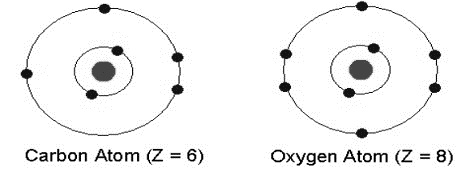Above 10 electrons, the 2nd shell encloses 8 electrons and is full. For the elements beyond (starting via sodium, Z = 11), the last electrons therefore sit in the 3rd shell, as shown here for sodium and chlorine (Z = 17):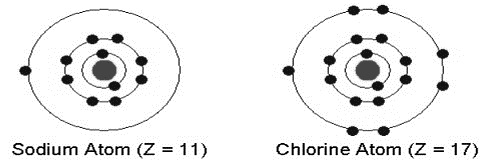This structural description leads naturally to a significant property of atoms, the octet rule: atoms have a strong tendency to lose, gain, or share electrons if this leads to them having a entire shell of electrons around them. In other terms, atoms prefer to have a total of 2, 10, or 18 electrons around them.

Ionic bonds

Elements in the 1st few columns of the periodic table have a few more electrons than expected via the octet rule: they thus lose the electrons in the outermost shells fairly easily. For instance, the alkali metals (group I), such as sodium (Na) or potassium (K), which have 11 and 19 electrons correspondingly, easily lose one electron to form mono-positive ions, Na+ and K+. Such ions have 10 and 18 electrons, correspondingly and they are quite stable according to the octet rule.

Elements in the last few columns of the periodic table have1, 2 or 3 fewer electrons than expected via the octet rule: they therefore gain electrons fairly easily. For instance, the halogens (group VII), such as fluorine (F) or chlorine (Cl), which have 9 and 17 electrons, respectively, easily gain one electron to form mono-negative ions, F-or Cl-. Such ions have 10 and 18 electrons, correspondingly.

Similarly, elements in group II form doubly positive ions such as Mg++or Ca++, and elements in group VI form doubly negative ions such as O--or S--. All such ions obey the octet rule and so are fairly stable.

Now, imagine what will take place when one sodium atom meets one chlorine atom: the sodium atom will lose one electron to provide Na+, and the chlorine atom will increase that electron to give Cl-. This can be symbolized schematically in the given way:Fig: Formation of Sodium Chloride by Ionic Bonding

The consequential ions, which have conflicting charges, will be attracted to one another, and will draw closer, until they 'touch'. This take place when the inner shell of electrons on the sodium ion (the smaller sphere) begins to overlap through the outer shell of electrons on the chloride anion (the bigger sphere). This pair of ions looks as this: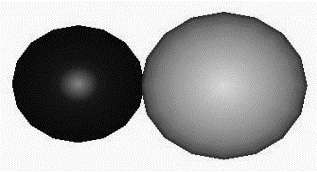It is possible to determine where the valence electrons are situated in this pair of ions. They are almost wholly positioned on the chlorine atom, as supposed: the sodium atom has lost its only valence (3s) electron, whereas chlorine has gained an electron and has the 3s23p6 valence configuration.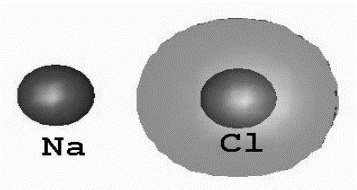NaCl, or sodium chloride, is though more complicated than this! This is since charge-charge interaction take place in all directions. Once a Na+ cation has attracted a Cl- anion in one direction, it can attract another in a different direction. So two pairs of ions such as above can come together to form a species through 4 ions in total, all situated so as to relate favorably by ions of opposite charge: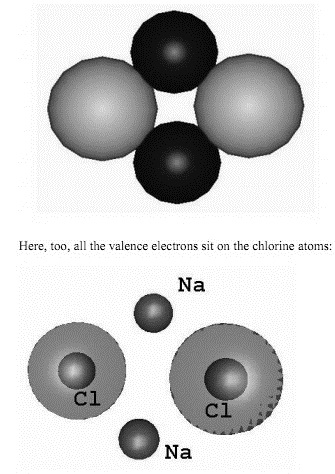And this need not stop here... The next step is to get 8 ions, 4 each of sodium and chlorine: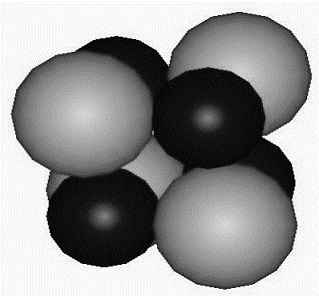The stable form of sodium chloride engages an extremely large number of NaCl units arranged in a lattice (or regular arrangement) millions of atoms across. Since the lattice is rigid, this means that one obtains a solid: the ions don't shift much by respect to one another. As well, since atoms are so little, even a small crystal of salt will have billions of sodium chloride units in it! The ions are organized so that each positive (sodium) ion is close to many negative (chloride) ions, as revealed in Figure below: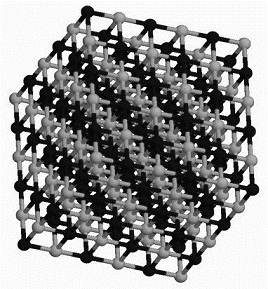Fig: The Arrangement of Ions such that each Positive (sodium) ion is close to many Negative (chloride) ions

Such pairs of ions in close contact are shown through lines joining them. Such lines demonstrate the strong ionic bond between ions of opposite charge which are subsequently to each other.

Though, we should remember that such close contacts aren't the similar as covalent bonds - there is no pair of electrons shared between the 2 atoms which are joined via the 2 lines. As well, there is several ionic bonding between ions which are additional away from each other - ions of reverse charge always attract each other, though far they are from each other. However, the force holding them together is hugest when they are close mutually. The lines attaching ions in this lattice (and others below) are there to make it easier to detect the pairs of ions in close contact by each other.

Remember - atoms are very small! The distance between a sodium ion and its closest to chloride ion neighbors is about 3 ten-millionths of a millimeter (3 Å or 3 x10-10 m). Imagine a cubic grain of salt by edges which are 3 tenths of a millimeter (3 x10-4 m) long. That implies that there will be a line of about a million (106) ions along each edge. And the grain will contain 106 x106 x 106(1018) ions in total.

All ionic compounds adopt a similar 3-dimensional structure in which the ions are close to many ions of the conflicting charge. As another instance, let us consider a salt by a divalent (doubly positive) ion, for instance calcium fluoride, CaF2. This accepts the structure following below (the calcium atoms are exposed as huge spheres; the fluorine atoms are smaller):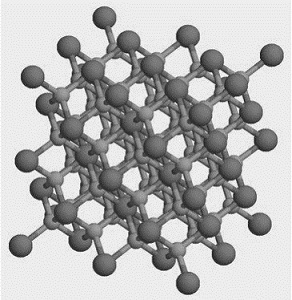Fig: 3-Dimensional Structure of Calcium Fluoride

Experienced chemists can frequently expect the structure that a given ionic species will accept, based on the nature of the ions included. This means that it is often likely to design ionic compounds having definite well-defined and attractive properties. As an instance, chemists have been able to make high-temperature superconductors, such as the complicated ionic compound, YBa2Cu3O4. This solid conducts electricity by no opposition at all at low temperature (below ~ -100 degrees centigrade). Preceding superconductors only had this property at much lower temperatures. The lack of resistance makes superconductors extremely helpful in a no of technological applications - for example in designing high-speed trains that levitate above the track!

Energetics of Ionic Bonding

We cannot easily measure the strength of an ionic bond, because ionic compounds do not break apart into gaseous ions:

NaCl(s)    →      Na+(g) + Cl-(g) (will not occur!)

Born-Haber cycles are used to estimate the strength of "ionic bonds" in compounds such as NaCl. In a Born-Haber cycle, we carry out the given sequence of reactions:

Elements → Gaseous atoms   →   Gaseous ions → Compound

(In their atoms ions standard states)

The energy of each step except the last one can be measured experimentally. In addition, we can measure the energy of the single-step reaction below (this is the "heat of formation" of the compound):

Elements (in their standard states)     →    Compound

By Hess's law, the energies of the reactions in the first sequence must add up to the heat of formation (the energy of the single-step reaction). This basic concept is not hard. The difficulty is in keeping the details straight. Ionic compounds contain two (or more) elements, each of which must be converted to gaseous atoms and then to gaseous ions. For example, here are the steps required to convert elemental calcium (a solid at room temperature) to gaseous calcium ions:

Sublime the solid calcium (convert it to a gas): Ca(s)   →    Ca(g)

Remove one electron from each atom:             Ca(g)    →    Ca+(g) + e-

Remove a second electron from each atom:     Ca+ (g) →   Ca2+ (g) + e-

Non-metals often form covalent molecules. If so, you must break the covalent bond as part of this process. Here are the steps required to convert elemental bromine (a diatomic liquid at room temperature) to gaseous bromide ions:

Vaporize the liquid bromine (convert it to a gas): Br2(l)   →   Br2(g)

Break the covalent bond in Br2:  Br2(g)  →  2Br(g)

Add an electron to each atom:    Br(g) + e-  →  Br- (g)

Your job in sorting out a Born-Haber cycle has two parts. The first is to be able to figure out exactly what reactions must occur when you convert the original element to a monatomic gas.

The 2nd is to know how to recognize the energy of each reaction kind.

The reactions we might see in a Born-Haber cycle include:

i. Heat of sublimation (ΔHsubl):  this is the energy required to convert a solid to a gas. For metals and most solid non-metals, sublimation creates a monatomic gas. For iodine (which is diatomic), sublimation creates I2(g).

Na(s)   →   Na(g)        S(s)   →   S(g)          I2(s)   →   I2(g)

Heats of sublimation are always positive numbers.

ii. Heat of vaporization (ΔHvap):  This is the energy required to change a liquid to a gas. The only elements for which this will approach into play are bromine and mercury that are liquids at room temperature. For bromine, vaporization produces Br2(g).

Hg(l)  → Hg(g)                        Br2(l)   →   Br2(g)

Heats of vaporization are always positive numbers.

iii. Bond dissociation energy (BDE, or ΔHBDE):  This is the energy required to break a covalent bond. Bond dissociation energies only come into play for the diatomic nonmetals (H2, N2, O2, F2, Cl2, Br2, I2 and At2). In such cases, sublimation or vaporization provides us diatomic molecules, not individual atoms, so we must as well break the covalent bonds in the gaseous form.

H2(g)   →  2 H(g)                                       N2(g)   →  2N(g)

Bond dissociation energies are always positive numbers.

iv. Ionisation energy (IE, or ΔHIE):  This is the energy required to remove one electron from a gaseous atom. Since many ionic compounds contain metals that have vanished two or more electrons, we often need to consider two or more consecutive ionisation energies. For instance, if we require making aluminium ions, we must eliminate 3 electrons from Al(g), so we must consider the 1st three ionisation energies of aluminium:

Al(g)   →     Al+ (g) + e   ΔH = 'first ionization energy' (IE1)

Al+(g)   →    Al2+ (g) + e-   ΔH = 'second ionization energy' (IE2)

Al2+(g)   →    Al3+ (g) + e-   ΔH = 'third ionization energy' (IE3)

Ionisation energies are always positive numbers, and they amplify as we eliminate more electrons (so IE1 < IE2 < IE3 for any given element).

v. Electron affinity (EA, or ΔHEA):  This is the energy absorbed or liberated when we add one electron to a gaseous atom. In common, only the 1st electron affinity can be computed directly, because negative ions repel electrons. Though, the second (and third, if necessary) electron affinity can be estimated using a variation on the Born-Haber cycle. Here are the reactions that must be considered if we require building oxide ions.

O(g) + e-     →   O-(g)     ΔH = 'first electron affinity' (EA1, or simply EA)

O-(g) + e-    →   O2-(g)   ΔH = 'second electron affinity' (EA2)

The 1st electron affinity is generally a negative number (a few elements have positive EA's). The second EA (and beyond) is always positive.

vi. Crystal lattice energy (CLE, or ΔHCLE): this is the energy released when gaseous ions are converted into the solid ionic compound. For example, the lattice energy of aluminium fluoride corresponds to the given reaction:

Al3+(g) + 3F-(g)  →   AlF3(s)     ΔH = crystal lattice energy of AlF3

Lattice energies are always huge negative numbers, ranging from around -600 to-13,000 kJ/mol.

vii. Heat of formation (ΔHf): This is the energy absorbed or liberated when we make an ionic compound from its constituent elements (as they usually appear at room temperature and 1 atmospheric pressure). For aluminium fluoride, the analogous reaction is:

Al(s) + 1½ F2(g)    →    AlF3(s)

Heats of formation are practically always negative numbers for ionic compounds.

The diagram below is the Born-Haber cycle for the formation of an ionic compound from the reaction of an alkali metal (Li, Na, K, Rb, Cs) through a gaseous halogen (F2, Cl2). The Born-Haber thermochemical cycle is called after the 2 German physical chemists, Max Born and Fritz Haber, who 1st utilized it in the year 1919.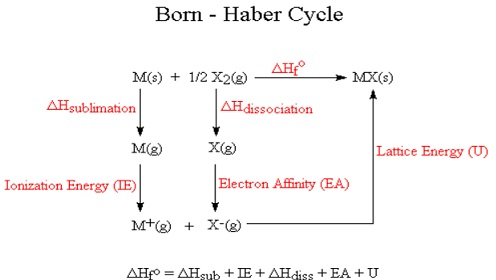Fig: The Born - Haber Cycle

The enthalpy change in the formation of an ionic lattice from the gaseous isolated sodium and chloride ions is -788 kJ/mole. That enthalpy change, which corresponds to the reaction Na+(g) +Cl-(g) → NaCl(s), is termed the lattice energy of the ionic crystal. Even though the lattice energy isn't directly measurable, there are diverse ways to estimate it from theoretical deliberations and several experimental values. For all recognized ionic crystals, the lattice energy has a large negative value. It is eventually the lattice energy of an ionic crystal that is responsible for the formation and stability of ionic crystal structures.

For sodium chloride, the Born - Haber cycle is: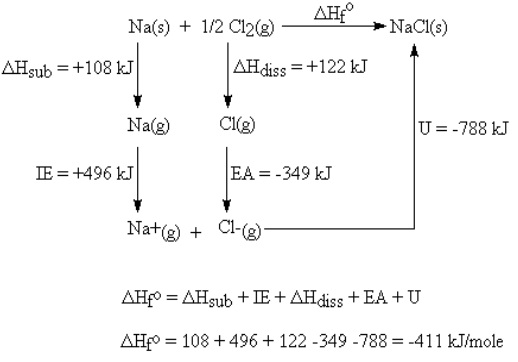The concept behind the Born-Haber cycle is based on Hess' Law, which follows from the 1st law of thermodynamics (law of conservation of energy).

Hess's Law:  If a reaction is carried out in a series of steps, the enthalpy change for the reaction will be equal to the sum of enthalpy transforms for the individual steps.

The Properties of Ionic Compounds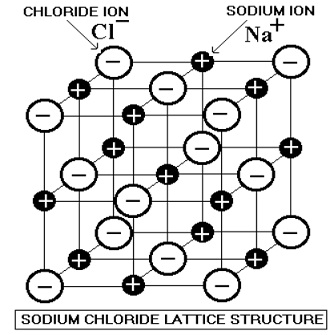Fig: The Lattice Structure of Sodium Chloride

i. The diagram above is classic of the giant ionic crystal structure of ionic compounds as sodium chloride and magnesium oxide.

ii. The alternate positive and negative ions in an ionic solid are assembled in an orderly way in a giant ionic lattice structure.

iii. The ionic bond is the strong electrical attraction between the positive and negative ions next to each other in the lattice.

iv. The bonding widens throughout the crystal in all directions.

v. Salts and metal oxides are typical ionic compounds.

vi. This strong bonding force is the basis of the hard structure (if brittle) and high melting and boiling points, so they aren't extremely volatile.

vii. A relatively huge amount of energy is required to melt or boil ionic compounds. The bigger the charges on the ions the stronger the bonding attraction e.g. magnesium oxide Mg2+O2- has a higher melting point than sodium chloride Na+Cl-.

viii. Unlike covalent molecules, ALL ionic compounds are crystalline solids at room temperature.

ix. They are hard but brittle, when stressed the bonds are broken along planes of ions that shear away. They are NOT malleable like metals.

x. Many ionic compounds are soluble in water, but not all, so don't build assumptions. Salts can melt in water since the ions can divide and become encircled via water molecules that weakly bond to the ions. This decreases the attractive forces between the ions, preventing the crystal structure to exist. Evaporating the water from a salt solution will eventually allow the ionic crystal lattice to reform.

xi. The solid crystals don't conduct electricity since the ions aren't free to move to carry an electric current. However, if the ionic compound is melted or dissolved in water, the liquid will now conduct electricity, as the ion particles are now free.

Tutorsglobe: A way to secure high grade in your curriculum (Online Tutoring)